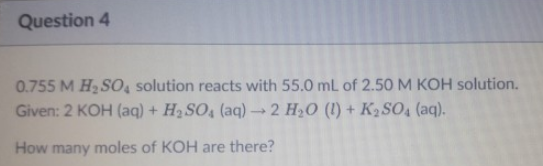# Problem: 0.755 M H2SO4 solution reacts with 55.0 mL of 2.50 M KOH solution. Given: 2KOH (aq) + H2SO4 (aq) → 2H2O (l) + K2SO4 (aq). How many moles of KOH are there?

###### FREE Expert Solution
83% (407 ratings)###### Problem Details

0.755 M H2SO4 solution reacts with 55.0 mL of 2.50 M KOH solution.

Given: 2KOH (aq) + H2SO4 (aq) → 2H2O (l) + K2SO4 (aq).

How many moles of KOH are there?